Imagine that you need to compute e^0.4 but you have no calculator or other aid to enable you to compute it exactly, only paper and pencil. Y

Question

Imagine that you need to compute e^0.4 but you have no calculator or other aid to enable you to compute it exactly, only paper and pencil. You decide to use a third-degree Taylor polynomial expanded around x =0. Use the fact that e^0.4 < e < 3 and the Error Bound for Taylor Polynomials to find an upper bound for the error in your approximation.

|error| <= _________

in progress 0
6 months 2021-07-25T17:59:54+00:00 1 Answers 1 views 0

0.0032

Step-by-step explanation:

We need to compute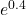by the help of third-degree Taylor polynomial that is expanded around at x = 0.

Given :< e < 3

Therefore, the Taylor’s Error Bound formula is given by :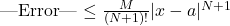, where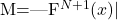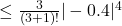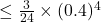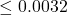Therefore, |Error| ≤ 0.0032